# cs231n 2019. A1, part 4. Two-layer net#### Solution by Yury Kashnitsky (@yorko)

In this exercise we will develop a neural network with fully-connected layers to perform classification, and test it out on the CIFAR-10 dataset.

In :
# A bit of setup

import numpy as np
import matplotlib.pyplot as plt

from cs231n.classifiers.neural_net import TwoLayerNet

%matplotlib inline
plt.rcParams['figure.figsize'] = (16.0, 12.0) # set default size of plots
plt.rcParams['image.interpolation'] = 'nearest'
plt.rcParams['image.cmap'] = 'gray'

def rel_error(x, y):
""" returns relative error """
return np.max(np.abs(x - y) / (np.maximum(1e-8, np.abs(x) + np.abs(y))))


We will use the class TwoLayerNet in the file cs231n/classifiers/neural_net.py to represent instances of our network. The network parameters are stored in the instance variable self.params where keys are string parameter names and values are numpy arrays. Below, we initialize toy data and a toy model that we will use to develop your implementation.

In :
# Create a small net and some toy data to check your implementations.
# Note that we set the random seed for repeatable experiments.

input_size = 4
hidden_size = 10
num_classes = 3
num_inputs = 5

def init_toy_model():
np.random.seed(0)
return TwoLayerNet(input_size, hidden_size, num_classes, std=1e-1)

def init_toy_data():
np.random.seed(1)
X = 10 * np.random.randn(num_inputs, input_size)
y = np.array([0, 1, 2, 2, 1])
return X, y

net = init_toy_model()
X, y = init_toy_data()


# Forward pass: compute scores¶

Open the file cs231n/classifiers/neural_net.py and look at the method TwoLayerNet.loss. This function is very similar to the loss functions you have written for the SVM and Softmax exercises: It takes the data and weights and computes the class scores, the loss, and the gradients on the parameters.

Implement the first part of the forward pass which uses the weights and biases to compute the scores for all inputs.

In :
scores = net.loss(X)
print(scores)
print()
print('correct scores:')
correct_scores = np.asarray([
[-0.81233741, -1.27654624, -0.70335995],
[-0.17129677, -1.18803311, -0.47310444],
[-0.51590475, -1.01354314, -0.8504215 ],
[-0.15419291, -0.48629638, -0.52901952],
[-0.00618733, -0.12435261, -0.15226949]])
print(correct_scores)
print()

# The difference should be very small. We get < 1e-7
print('Difference between your scores and correct scores:')
print(np.sum(np.abs(scores - correct_scores)))

Your scores:
[[-0.81233741 -1.27654624 -0.70335995]
[-0.17129677 -1.18803311 -0.47310444]
[-0.51590475 -1.01354314 -0.8504215 ]
[-0.15419291 -0.48629638 -0.52901952]
[-0.00618733 -0.12435261 -0.15226949]]

correct scores:
[[-0.81233741 -1.27654624 -0.70335995]
[-0.17129677 -1.18803311 -0.47310444]
[-0.51590475 -1.01354314 -0.8504215 ]
[-0.15419291 -0.48629638 -0.52901952]
[-0.00618733 -0.12435261 -0.15226949]]

Difference between your scores and correct scores:
3.6802720496109664e-08


# Forward pass: compute loss¶

In the same function, implement the second part that computes the data and regularizaion loss.

In :
loss, _ = net.loss(X, y, reg=0.05)
correct_loss = 1.30378789133

# should be very small, we get < 1e-12
print('Difference between your loss and correct loss:')
print(np.sum(np.abs(loss - correct_loss)))

Difference between your loss and correct loss:
1.794120407794253e-13


# Backward pass¶

Implement the rest of the function. This will compute the gradient of the loss with respect to the variables W1, b1, W2, and b2. Now that you (hopefully!) have a correctly implemented forward pass, you can debug your backward pass using a numeric gradient check:

In :
from cs231n.gradient_check import eval_numerical_gradient

# Use numeric gradient checking to check your implementation of the backward pass.
# If your implementation is correct, the difference between the numeric and
# analytic gradients should be less than 1e-8 for each of W1, W2, b1, and b2.

loss, grads = net.loss(X, y, reg=0.05)

# these should all be less than 1e-8 or so
f = lambda W: net.loss(X, y, reg=0.05)

W2 max relative error: 3.440708e-09
b2 max relative error: 1.276034e-10
W1 max relative error: 3.561318e-09
b1 max relative error: 3.833134e-09


# Train the network¶

To train the network we will use stochastic gradient descent (SGD), similar to the SVM and Softmax classifiers. Look at the function TwoLayerNet.train and fill in the missing sections to implement the training procedure. This should be very similar to the training procedure you used for the SVM and Softmax classifiers. You will also have to implement TwoLayerNet.predict, as the training process periodically performs prediction to keep track of accuracy over time while the network trains.

Once you have implemented the method, run the code below to train a two-layer network on toy data. You should achieve a training loss less than 0.2.

In :
net = init_toy_model()
stats = net.train(X, y, X, y,
learning_rate=1e-1, reg=5e-6, batch_size=1,
num_iters=100, verbose=False)

print('Final training loss: ', stats['loss_history'][-1])

# plot the loss history
plt.plot(stats['loss_history'])
plt.xlabel('iteration')
plt.ylabel('training loss')
plt.title('Training Loss history')
plt.show()

Final training loss:  0.008116963954598025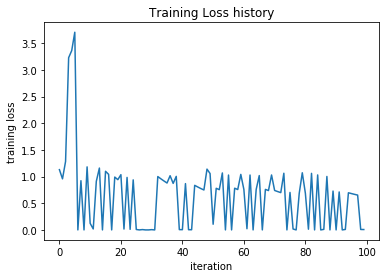Now that you have implemented a two-layer network that passes gradient checks and works on toy data, it's time to load up our favorite CIFAR-10 data so we can use it to train a classifier on a real dataset.

In :
from cs231n.data_utils import load_CIFAR10

PATH_TO_CIFAR = '/home/yorko/data/cifar-10-batches-py/'

def get_CIFAR10_data(num_training=49000, num_validation=1000, num_test=1000):
"""
Load the CIFAR-10 dataset from disk and perform preprocessing to prepare
it for the two-layer neural net classifier. These are the same steps as
we used for the SVM, but condensed to a single function.
"""
# Load the raw CIFAR-10 data
X_train, y_train, X_test, y_test = load_CIFAR10(PATH_TO_CIFAR)

# Subsample the data
mask = list(range(num_training, num_training + num_validation))

# Normalize the data: subtract the mean image
mean_image = np.mean(X_train, axis=0)
X_train -= mean_image
X_val -= mean_image
X_test -= mean_image

# Reshape data to rows
X_train = X_train.reshape(num_training, -1)
X_val = X_val.reshape(num_validation, -1)
X_test = X_test.reshape(num_test, -1)

return X_train, y_train, X_val, y_val, X_test, y_test

# Invoke the above function to get our data.
X_train, y_train, X_val, y_val, X_test, y_test = get_CIFAR10_data()
print('Train data shape: ', X_train.shape)
print('Train labels shape: ', y_train.shape)
print('Validation data shape: ', X_val.shape)
print('Validation labels shape: ', y_val.shape)
print('Test data shape: ', X_test.shape)
print('Test labels shape: ', y_test.shape)

Train data shape:  (49000, 3072)
Train labels shape:  (49000,)
Validation data shape:  (1000, 3072)
Validation labels shape:  (1000,)
Test data shape:  (1000, 3072)
Test labels shape:  (1000,)


# Train a network¶

To train our network we will use SGD with momentum. In addition, we will adjust the learning rate with an exponential learning rate schedule as optimization proceeds; after each epoch, we will reduce the learning rate by multiplying it by a decay rate.

In :
input_size = 32 * 32 * 3
hidden_size = 50
num_classes = 10
net = TwoLayerNet(input_size, hidden_size, num_classes)

# Train the network
stats = net.train(X_train, y_train, X_val, y_val,
num_iters=1000, batch_size=256,
learning_rate=1e-3, learning_rate_decay=0.95,
reg=0.25, verbose=True)

# Predict on the validation set
val_acc = (net.predict(X_val) == y_val).mean()
print('Validation accuracy: ', val_acc)

iteration 0 / 1000: loss 2.302944
iteration 100 / 1000: loss 1.860528
iteration 200 / 1000: loss 1.804372
iteration 300 / 1000: loss 1.635850
iteration 400 / 1000: loss 1.614847
iteration 500 / 1000: loss 1.557273
iteration 600 / 1000: loss 1.599602
iteration 700 / 1000: loss 1.540287
iteration 800 / 1000: loss 1.544831
iteration 900 / 1000: loss 1.585729
Validation accuracy:  0.474


# Debug the training¶

With the default parameters we provided above, you should get a validation accuracy of about 0.29 on the validation set. This isn't very good.

One strategy for getting insight into what's wrong is to plot the loss function and the accuracies on the training and validation sets during optimization.

Another strategy is to visualize the weights that were learned in the first layer of the network. In most neural networks trained on visual data, the first layer weights typically show some visible structure when visualized.

In :
# Plot the loss function and train / validation accuracies
plt.subplot(2, 1, 1)
plt.plot(stats['loss_history'])
plt.title('Loss history')
plt.xlabel('Iteration')
plt.ylabel('Loss')

plt.subplot(2, 1, 2)
plt.plot(stats['train_acc_history'], label='train')
plt.plot(stats['val_acc_history'], label='val')
plt.title('Classification accuracy history')
plt.xlabel('Epoch')
plt.ylabel('Clasification accuracy')
plt.legend()
plt.show()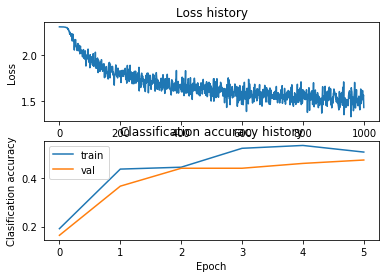In :
from cs231n.vis_utils import visualize_grid

# Visualize the weights of the network

def show_net_weights(net):
W1 = net.params['W1']
W1 = W1.reshape(32, 32, 3, -1).transpose(3, 0, 1, 2)
plt.gca().axis('off')
plt.show()

show_net_weights(net)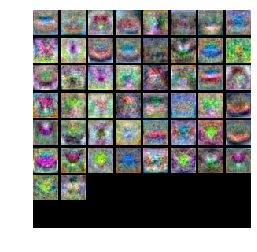What's wrong?. Looking at the visualizations above, we see that the loss is decreasing more or less linearly, which seems to suggest that the learning rate may be too low. Moreover, there is no gap between the training and validation accuracy, suggesting that the model we used has low capacity, and that we should increase its size. On the other hand, with a very large model we would expect to see more overfitting, which would manifest itself as a very large gap between the training and validation accuracy.

Tuning. Tuning the hyperparameters and developing intuition for how they affect the final performance is a large part of using Neural Networks, so we want you to get a lot of practice. Below, you should experiment with different values of the various hyperparameters, including hidden layer size, learning rate, numer of training epochs, and regularization strength. You might also consider tuning the learning rate decay, but you should be able to get good performance using the default value.

Approximate results. You should be aim to achieve a classification accuracy of greater than 48% on the validation set. Our best network gets over 52% on the validation set.

Experiment: You goal in this exercise is to get as good of a result on CIFAR-10 as you can, with a fully-connected Neural Network. For every 1% above 52% on the Test set we will award you with one extra bonus point. Feel free implement your own techniques (e.g. PCA to reduce dimensionality, or adding dropout, or adding features to the solver, etc.).

Explain your hyperparameter tuning process below.

$\color{blue}{\textit Your Answer:}$ Typically you start with a wide range of hyperparameters, and then narrow down the search space around good values. You need to make sure that you don't get optimal loss (or accuracy) values with extreme values of hyperparameters, otherwise you need to change the range of values to try. That's how I first defined good hidden size and a range of learning rate values. Then learning rate decay and reqularization strength. Not all history of experiments is shown in this notebook. Below we iterate over the final grid of hyperparameters.

In :
from tqdm import tqdm_notebook

In :
%%time
best_net = None # store the best model into this
results = {}
best_val_acc = -1
# some params are fixed, others are varied
num_iters = 2000
hidden_size = 150
batch_size = 256
learning_rate_decay = 0.95
learning_rates = np.linspace(1e-4, 1e-3, 5)
regularization_strengths = np.linspace(1e-6, 1e-4, 5)

#################################################################################
# TODO: Tune hyperparameters using the validation set. Store your best trained  #
# model in best_net.                                                            #
#                                                                               #
# To help debug your network, it may help to use visualizations similar to the  #
# ones we used above; these visualizations will have significant qualitative    #
# differences from the ones we saw above for the poorly tuned network.          #
#                                                                               #
# Tweaking hyperparameters by hand can be fun, but you might find it useful to  #
# write code to sweep through possible combinations of hyperparameters          #
# automatically like we did on the previous exercises.                          #
#################################################################################
best_params = None
for lr in tqdm_notebook(learning_rates):
for reg in tqdm_notebook(regularization_strengths):
print('lr: {}, reg: {}'.format(lr, reg))
net = TwoLayerNet(input_size, hidden_size, num_classes)
stats = net.train(X_train, y_train, X_val, y_val,
num_iters=num_iters, batch_size=batch_size,
learning_rate=lr, learning_rate_decay=learning_rate_decay,
reg=reg, verbose=False)
y_train_pred = net.predict(X_train)
train_acc = np.mean(y_train == y_train_pred)

val_acc = (net.predict(X_val) == y_val).mean()
print('Validation accuracy: ', val_acc)
results[(lr, reg)] = val_acc
if val_acc > best_val_acc:
best_val_acc = val_acc
best_net = net
best_params = (lr, reg)
#################################################################################
#                               END OF YOUR CODE                                #
#################################################################################

lr: 0.0001, reg: 1e-06
Validation accuracy:  0.366
lr: 0.0001, reg: 2.5750000000000002e-05
Validation accuracy:  0.366
lr: 0.0001, reg: 5.05e-05
Validation accuracy:  0.37
lr: 0.0001, reg: 7.525e-05
Validation accuracy:  0.364
lr: 0.0001, reg: 0.0001
Validation accuracy:  0.366

lr: 0.000325, reg: 1e-06
Validation accuracy:  0.47
lr: 0.000325, reg: 2.5750000000000002e-05
Validation accuracy:  0.467
lr: 0.000325, reg: 5.05e-05
Validation accuracy:  0.481
lr: 0.000325, reg: 7.525e-05
Validation accuracy:  0.465
lr: 0.000325, reg: 0.0001
Validation accuracy:  0.469

lr: 0.00055, reg: 1e-06
Validation accuracy:  0.509
lr: 0.00055, reg: 2.5750000000000002e-05
Validation accuracy:  0.505
lr: 0.00055, reg: 5.05e-05
Validation accuracy:  0.504
lr: 0.00055, reg: 7.525e-05
Validation accuracy:  0.497
lr: 0.00055, reg: 0.0001
Validation accuracy:  0.507

lr: 0.0007750000000000001, reg: 1e-06
Validation accuracy:  0.51
lr: 0.0007750000000000001, reg: 2.5750000000000002e-05
Validation accuracy:  0.515
lr: 0.0007750000000000001, reg: 5.05e-05
Validation accuracy:  0.498
lr: 0.0007750000000000001, reg: 7.525e-05
Validation accuracy:  0.522
lr: 0.0007750000000000001, reg: 0.0001
Validation accuracy:  0.499

lr: 0.001, reg: 1e-06
Validation accuracy:  0.515
lr: 0.001, reg: 2.5750000000000002e-05
Validation accuracy:  0.521
lr: 0.001, reg: 5.05e-05
Validation accuracy:  0.52
lr: 0.001, reg: 7.525e-05
Validation accuracy:  0.52
lr: 0.001, reg: 0.0001
Validation accuracy:  0.523

CPU times: user 37min 25s, sys: 4.09 s, total: 37min 29s
Wall time: 6min 14s

In :
best_params, best_val_acc

Out:
((0.001, 0.0001), 0.523)
In :
%%time
best_net = TwoLayerNet(input_size, hidden_size, num_classes)
stats = best_net.train(X_train, y_train, X_val, y_val,
num_iters=3000, batch_size=batch_size,
learning_rate=best_params, learning_rate_decay=learning_rate_decay,
reg=best_params, verbose=True)

iteration 0 / 3000: loss 2.302631
iteration 100 / 3000: loss 1.942662
iteration 200 / 3000: loss 1.694240
iteration 300 / 3000: loss 1.642821
iteration 400 / 3000: loss 1.634989
iteration 500 / 3000: loss 1.581651
iteration 600 / 3000: loss 1.482083
iteration 700 / 3000: loss 1.443788
iteration 800 / 3000: loss 1.483015
iteration 900 / 3000: loss 1.384776
iteration 1000 / 3000: loss 1.511768
iteration 1100 / 3000: loss 1.275055
iteration 1200 / 3000: loss 1.365429
iteration 1300 / 3000: loss 1.305279
iteration 1400 / 3000: loss 1.355764
iteration 1500 / 3000: loss 1.278184
iteration 1600 / 3000: loss 1.154499
iteration 1700 / 3000: loss 1.172329
iteration 1800 / 3000: loss 1.322811
iteration 1900 / 3000: loss 1.401773
iteration 2000 / 3000: loss 1.238886
iteration 2100 / 3000: loss 1.155777
iteration 2200 / 3000: loss 1.236830
iteration 2300 / 3000: loss 1.149130
iteration 2400 / 3000: loss 1.168785
iteration 2500 / 3000: loss 1.139603
iteration 2600 / 3000: loss 1.160867
iteration 2700 / 3000: loss 1.064185
iteration 2800 / 3000: loss 1.009131
iteration 2900 / 3000: loss 1.114445
CPU times: user 2min 5s, sys: 160 ms, total: 2min 5s
Wall time: 21 s

In :
# Plot the loss function and train / validation accuracies
plt.subplot(2, 1, 1)
plt.plot(stats['loss_history'])
plt.title('Loss history')
plt.xlabel('Iteration')
plt.ylabel('Loss')

plt.subplot(2, 1, 2)
plt.plot(stats['train_acc_history'], label='train')
plt.plot(stats['val_acc_history'], label='val')
plt.title('Classification accuracy history')
plt.xlabel('Epoch')
plt.ylabel('Clasification accuracy')
plt.legend()
plt.show()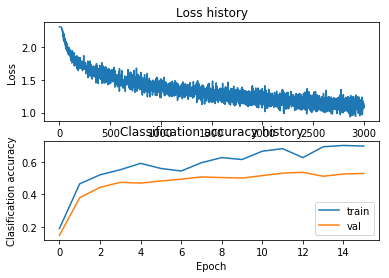In :
# visualize the weights of the best network
show_net_weights(best_net)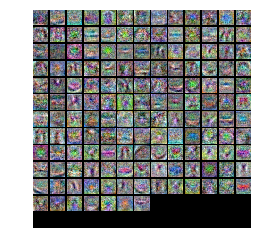# Run on the test set¶

When you are done experimenting, you should evaluate your final trained network on the test set; you should get above 48%.

We will give you extra bonus point for every 1% of accuracy above 52%.

In :
test_acc = (best_net.predict(X_test) == y_test).mean()
print('Test accuracy: ', test_acc)

Test accuracy:  0.525


Inline Question

Now that you have trained a Neural Network classifier, you may find that your testing accuracy is much lower than the training accuracy. In what ways can we decrease this gap? Select all that apply.

1. Train on a larger dataset.
$\color{blue}{\textit Your Answer:}$ 2
$\color{blue}{\textit Your Explanation:}$ With validation curves, we see that validation accuracy goes on increasing, thus the algorithm is underfitted.